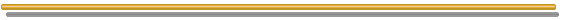# FAQ Number 13

## How to Write a Risk-Neutral Process for the Mean-Reversion Case?

There are two equivalent ways to do this:

1. First method, like that showed for the Geometric Brownian case, is just to substitute the real drift a of the process by the risk-neutral drift r - d. The difference in mean-reversion case is that the dividend-yield d (or convenience yield for commodities) is not constant, it is a function of P.
2. Second method is to subtract a risk premium ls from the real drift of the process, where l is the market price of risk for the underlying asset.

Consider the following mean-reversion process, an arithmetic Ornstein-Uhlenbeck process, that has been largely used in financial and real options applications:

dx = h (m - x) dt + s dz

For oil prices P, let x = ln(P), as in Schwartz (1997, his "Model 1"). So, we can write the same equation for the logarithm of oil prices following a mean-reverting process:

d(lnP) = h (m - lnP) dt + s dz

The drift a here is the mean-reversion term, a = h (m - x). For the risk-neutral process, the first method indicate that we can substitute this for the risk-neutralized drift:

a* = r - d

But the risk-adjusted discount rate m is the total expected return, which is the sum of capital gain rate with the dividend rate, that is, m = a + d. So, we can write the equation of d as:

d = m - a , hence: d = m - h (m - x)

The risk-neutral drift becomes:

a* = r - m + h (m - x)

The stochastic risk-neutral equation finally becomes:

dx = [r - m + h (m - x)] dt + s dz*

For the oil prices case:

d(lnP) = [ h (m - lnP) - (m -r) ] dt + s dz* ............. (Eq.1)

Where dz* is the Wiener increment under risk-neutral probability (or under martingale measure as the mathematicians prefer).
This format of risk-neutral differential equation is found in Dixit & Pindyck (e.g., see mean-reversion in chapters 5 and 12).

We can also rewrite the equation 1 by rearranging the terms:

d(lnP) = h [m - [(m - r)/h] - lnP] dt + s dz* ............. (Eq.1a)

Note that m - r is the risk-premium for the underlying asset x. The comparison between both drifts indicates that the passage from the real process to the risk-neutral one, can be viewed as subtracting the normalized risk-premium (( m - r )/h) from the long run mean level m.

Let us see the second method, which we can find other format for the same Eq.1. The idea here is to subtract a risk premium ls from the real drift of the process a. Of course r - d = a - ls, but let us subtract the premium risk directly from the stochastic equation, in order to get a risk-neutral stochastic process:

dx = [h (m - x) - ls] dt + s dz*

So, is easy to see that:

dx = h [(m - ls/h)- x] dt + s dz*

Or, in oil prices terms:

d(lnP) = h [(m - ls/h) - lnP] dt + s dz* ..............(Eq.2)

This equation is equal to the Eqs.1 and 1a, but using other parameters. The interpretation again is that the risk-neutral process difference from the real process is just an adjustment in the equilibrium (or long-run mean) level m, which the process reverts.
That is, by subtracting ls/h in the equilibrium level m, we get the risk-neutralized process for mean-reversion.

This second method interpretation is drawn from a similar case found in the Bjerksund & Ekern (1993) paper and Schwartz (1997, model1).

For additional information, see the page on simulation of stochastic processes.Go to the Next FAQ: 14) How to combine technical and market uncertainties into a dynamic real options model?

Back to the FAQ's ListBack to Contents